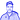# Notes on

May 25, 2019

1. ## Computing with Language: Texts and Words

### 1.2 Getting Started with NLTK

``````import nltk

``from nltk.book import *``

### 1.3 Searching Text

What is a concordance view?
A concordance view displays every occurrence of a given word in its context (= preceding and following words).

``string.concordance('word')``

What other words appear in a similar range of contexts?

``text.similar('word')``

How to examine shared contexts between words?

``text.common_contexts(['word', 'writer'])``

What’s a dispersion plot? How to obtain it?
A dispersion plot displays the locations of a word in the text,
each stripe represents an instance of a word.

``text.dispersion_plot(['citizens', 'democracy', 'freedom', 'duties', 'America'])``

### 1.4 Counting Vocabulary

What’s a token?
A sequence of characters: a word and/or a punctuation symbol.

How to obtain the number of tokens?

``len(text)``

What’s the vocabulary of a text?
A vocabulary is the set of tokens contained in a text.

``set(text)``

How to sort an array in Python?

``sorted(array)``

What’s a word type?
A word considered to be an unique item within a given vocabulary.

How do you quantify the lexical richness of a text?
Divide the number of distinct words over the total number of words. The result is a percentage of distincts words.

``len(set(text)) / len(text)``

How to count occurrences of a specific word within a text?

``text.count('word')``

How to create a function in Python?

``````def function_x(param):
return true``````
1. ## A Closer Look at Python: Texts as Lists of Words

### 2.1 Lists

How to create a list in Python?

``[‘’, ‘’ etc.]``

How to concatenate lists in Python?

``[ ] + [ ]``

How to append an element to a list in Python?

``list.append(element)``

### 2.2 Indexing lists

What’s an index?
It’s the position of an item inside an array/list.

How to access an index from a value?

``text.index(value)``

How to access a value given an index?

``text[index]``

What is slicing?
It’s retrieving a subpart of an array/list.

``text[index1:index2] ; text[index1:] ; text[:index2]``

### 2.4 Strings

What’s a string?
Strings are lists of characters, so a string shares the same properties than a list.

How to convert a list to a string?

``' '.join(list)``

How to convert a string to a list?

``string.split()``
1. ## Computing with Language: Simple Statistics

### 3.1 Frequency Distributions

What is a frequency distribution?
It’s a matrix where each row represents the frequency of a vocabulary item in a given text.

``fdist = FreqDist(text)``

How to get the most frequent tokens?

``fdist.most_common(<number of tokens to get>)``

How to obtain a cumulative frequency plot?
A cumulative frequency plot tells us what proportion of a text is taken by the most common tokens:

``fdist.plot(50, cumulative=True)``

What’s an hapaxe?
An hapaxe is word that occur only once in a text. Hapaxes are considered as outliers in data analysis, and thus not generally useful.

``fdist.hapaxes()``

### 3.2 Fine-grained Selection of Words

How to operate a fine-grained word selection by word length and frequency?
Obtain words which are at least 7 character long and that appear at least 7 times in the text:

``w for w in set(text) if len(w) > 7 and fdist[w] > 7``

The result is useful to identify key words in a text content-wise.

### 3.3 Collocations and Bigrams

What’s a bigram?
A pair of words.

``list(bigrams(['more', 'is', 'said', 'than', 'done']))``

What is a collocation?
A sequence of words that occur together unusually often, and which are resistant to substitution with words that have similar meanings. A collocation is a frequent bigram.

``text.collocations()``

### 3.4 Counting Other Things

How to get the frequency distribution of the different word lengths?

``fdist = FreqDist(len(w) for w in text)``

How to obtain the max value in a list?

``fdist.max()``

How to access a given frequency in a frequency distribution?

``fdist.freq(frequency_index)``
1. ## Back to Python: making decisions and taking control

How to create an if statement in Python?

``````if len(word) < 5:
...    print('word length is less than 5')
elif token.istitle():
...     print(token, 'is a titlecase word')
else:
...     print(token, 'is punctuation')``````

How to create a loop in Python?

``````for word in ['Call', 'me', 'Ishmael', '.']:
...    print(word)``````

How to operate on every element of a loop?

``[function(w) for w in text]``
1. ## Automatic Natural Language Understanding

What is Word Sense Disambiguation?
It’s an area of NLP where we want to discover the intended meaning of a word in a given context.

What is Pronoun Resolution
It’s about detecting the subjects and objects of verbs, finding the antecedents of a word.

What is Anaphora Resolution?
It’s a part of pronoun resolution where we identify what a pronoun or noun refers to.

What is Semantic Role Labeling?
It’s about identifying how a noun relates to the verb. Also a part of Pronoun Resolution.

What is Text Alignment?
It’s a program automatically pairing up sentences. Once we have a million or more sentence pairs, we can detect corresponding words and phrases, and build a model that can be used for translating new text for example.

What is a Spoken Dialogue System?
It’s a pipeline of language understanding components to generate a speech answer to an audio question.

What is RTE (Recognizing Textual Entailment)?
It’s a challenge in language understanding where you try to automatically verify an hypothesis from statements given previously.Written by Basile Samel.